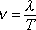The speed v of a wave is equal to the wavelength lambda of the wave divided by the period-of-oscillations T of the wave.

Note: The wave speed is predetermined by the characteristics of the medium (tension and mass-per-length in the case of a string). So this equation is saying that no matter what frequency wave is caused to travel in the medium, the ratio of the wavelength of the wave to the period of the wave will have one and the same value, namely the predetermined value of the wave speed.

Note: This equation is often written in terms of the frequency rather than the period as v = lambda times f, taking advantage of the fact that 1/T = f.